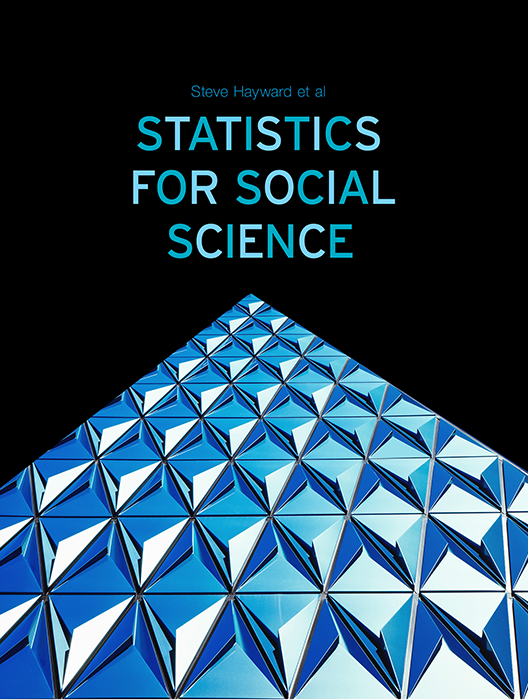# Statistics for Social Science

Statistics for Social Science takes a fresh approach to the introductory class. With learning check questions, embedded videos and interactive simulations, students engage in active learning as they read. An emphasis on real-world and academic applications help ground the concepts presented. Designed for students taking an introductory statistics course in psychology, sociology or any other social science discipline.

## What is a Top Hat Textbook?

Top Hat has reimagined the textbook – one that is designed to improve student readership through interactivity, is updated by a community of collaborating professors with the newest information, and accessed online from anywhere, at anytime.

• Top Hat Textbooks are built full of embedded videos, interactive timelines, charts, graphs, and video lessons from the authors themselves
• High-quality and affordable, at a significant fraction in cost vs traditional publisher textbooks

## Key features in this textbook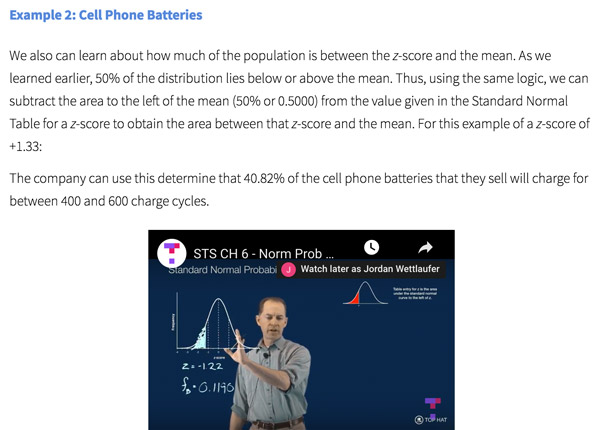Our Statistics for Social Science textbook allows students to manipulate data, visualize the effects discussed, and explore Lightboard videos that feature instructor explanations to reinforce concepts and calculations.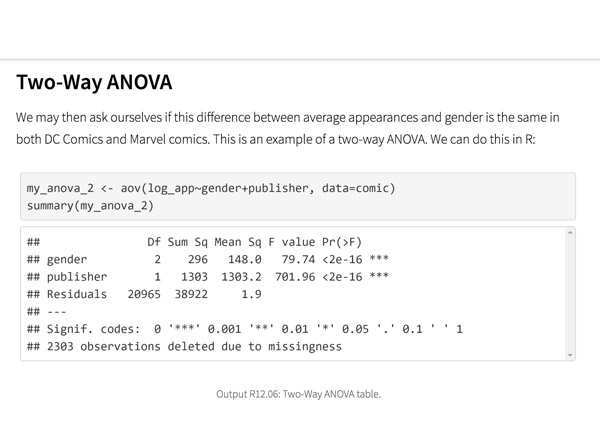Top Hat’s interactive offering includes a complementary module on using R software for data management, graphics, and conducting statistical analyses with examples and practice questions.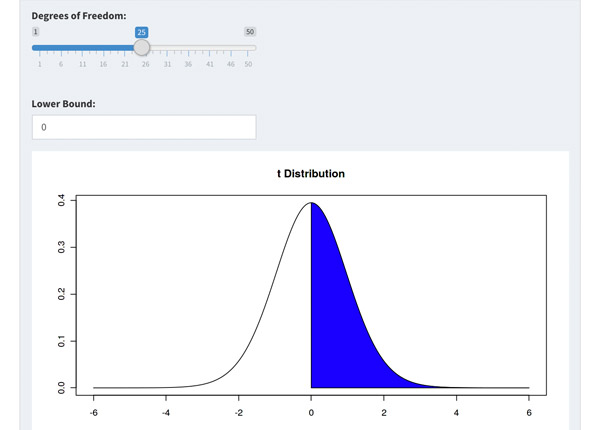Built-in assessment questions embedded throughout chapters so students can read a little, do a little, and test themselves to see what they know!

## Comparison of Social Sciences Textbooks

Consider adding Top Hat’s Statistics for Social Sciences textbook to your upcoming course. We’ve put together a textbook comparison to make it easy for you in your upcoming evaluation.

### Top Hat

Steve Hayward et al., Statistics for Social Sciences, Only one edition needed

### Pearson

Agresti, Statistical Methods for the Social Sciences, 5th Edition

### Cengage

Gravetter et al., Essentials of Statistics for The Behavioral Sciences, 9th Edition

### Sage

Gregory Privitera, Essentials Statistics for the Behavioral Sciences, 2nd Edition

### Pricing

Average price of textbook across most common format

#### $200.83 Hardcover print text only ####$239.95

Hardcover print text only

#### $92 Hardcover print text only ### Always up-to-date content, constantly revised by community of professors Content meets standard for Introduction to Anatomy & Physiology course, and is updated with the latest content ### In-Book Interactivity Includes embedded multi-media files and integrated software to enhance visual presentation of concepts directly in textbook Only available with supplementary resources at additional cost Only available with supplementary resources at additional cost Only available with supplementary resources at additional cost ### Customizable Ability to revise, adjust and adapt content to meet needs of course and instructor ### All-in-one Platform Access to additional questions, test banks, and slides available within one platform ## Pricing Average price of textbook across most common format ### Top Hat Steve Hayward et al., Statistics for Social Sciences, Only one edition needed #### Up to40-60%more affordable Lifetime access on any device ### Pearson Agresti, Statistical Methods for the Social Sciences, 5th Edition ####$200.83

Hardcover print text only

### Pearson

Gravetter et al., Essentials of Statistics for The Behavioral Sciences, 9th Edition

#### $239.95 Hardcover print text only ### Sage McConnell, Brue, Flynn, Principles of Microeconomics, 7th Edition ####$92

Hardcover print text only

## Always up-to-date content, constantly revised by community of professors

Constantly revised and updated by a community of professors with the latest content

### Top Hat

Steve Hayward et al., Statistics for Social Sciences, Only one edition needed

### Pearson

Agresti, Statistical Methods for the Social Sciences, 5th Edition

### Pearson

Gravetter et al., Essentials of Statistics for The Behavioral Sciences, 9th Edition

### Sage

Gregory Privitera, Essentials Statistics for the Behavioral Sciences, 2nd Edition

## In-book Interactivity

Includes embedded multi-media files and integrated software to enhance visual presentation of concepts directly in textbook

### Top Hat

Steve Hayward et al., Statistics for Social Sciences, Only one edition needed

### Pearson

Agresti, Statistical Methods for the Social Sciences, 5th Edition

### Pearson

Gravetter et al., Essentials of Statistics for The Behavioral Sciences, 9th Edition

### Sage

Gregory Privitera, Essentials Statistics for the Behavioral Sciences, 2nd Edition

## Customizable

Ability to revise, adjust and adapt content to meet needs of course and instructor

### Top Hat

Steve Hayward et al., Statistics for Social Sciences, Only one edition needed

### Pearson

Agresti, Statistical Methods for the Social Sciences, 5th Edition

### Pearson

Gravetter et al., Essentials of Statistics for The Behavioral Sciences, 9th Edition

### Sage

Gregory Privitera, Essentials Statistics for the Behavioral Sciences, 2nd Edition

## All-in-one Platform

### Top Hat

Steve Hayward et al., Statistics for Social Sciences, Only one edition needed

### Pearson

Agresti, Statistical Methods for the Social Sciences, 5th Edition

### Pearson

Gravetter et al., Essentials of Statistics for The Behavioral Sciences, 9th Edition

### Sage

Gregory Privitera, Essentials Statistics for the Behavioral Sciences, 2nd Edition

A lifelong learner, Steve focused on statistics and research methodology during his graduate training at the University of New Mexico. He later founded and served as CEO of the Center for Performance Technology, providing instructional design and training development support to larger client organizations throughout the United States. Steve is presently a lead faculty member for statistics at Rio Salado College in Tempe, Arizona.

## Explore this textbook

Read the fully unlocked textbook below, and if you’re interested in learning more, get in touch to see how you can use this textbook in your course today.

# Correlation and Regression

## Chapter Objectives

After completing this chapter, you will be able to:

• Identify the strength and direction of associations
• Construct and interpret scatterplots
• Calculate and interpret Pearson’s r and Spearman’s rho correlations
• Test the significance of correlation coefficients
• Understand explained/unexplained variation via the coefficient of determination
• Differentiate between correlation and causality
• Conduct simple linear regression between two variables

## What Are Correlation and Regression?

Correlation and regression are related descriptive statistical techniques that are often used in conjunction with one another. Using these techniques, we can quantify the strength and direction of an association between variables. When two random variables are associated, we say that they co-vary. As values on one variable change, the distribution of the second variable changes as well. For example, as the number of years of education changes, so does income. Regression is a logical extension of correlation in that it moves beyond describing the strength and direction of the association by making more specific predictions based on that association. In other words, for any given value of x (such as the number of years of education a person has attained), we can predict the corresponding value of y (such as the amount of income that person will receive).

Example

The concept of association took on an urgency in late 2015 when Latin American health authorities noticed a sudden spike in new cases (known as incidence in epidemiological terms) of a disease called microcephaly. Babies born with microcephaly have abnormally small heads and face a host of problems including intellectual disability and hearing/vision loss. It was strongly suspected that the increased incidence of microcephaly was caused by a recent outbreak of Zika virus, a mosquito-borne infection that has existed for decades but normally resolves itself without major complications. On February 1, 2016, the director of the World Health Organization declared the Zika virus to be a global public health emergency.

One thing that was crucial about their announcement, but not shown in our brief video clip, was their acknowledgement that the association between the increased number of Zika infections and the increased incidence of microcephaly had not yet been proven to be causal. Correlations are often merely coincidental. But given the severity of the birth defects and extent of their sudden increase in number, the WHO knew that the possibility of a causal relationship was strong enough to warrant urgent scientific exploration. By examining patterns between different events, correlation and regression analyses can help scientists make predictions about future events. Many of the "breaking research findings" that you encounter on a regular basis are the result of correlation and regression analyses, including fields as diverse as Medicine, Economics, Psychology, and more.

## Types of Correlation Coefficient

In practice, we tend to use the word "correlation" as shorthand for something more specific, known as a correlation coefficient. A correlation coefficient is a number that quantifies the strength and direction of an association between two variables. As such, correlations are very elegant; they take a pattern that we see in our data and summarize it in the form of a single, concise number between 1 and -1. You will be able to see what those numbers actually look like in more detail in the next section.

There is a variety of types of correlation coefficients, also known as measures of association. Choosing from among them can be very daunting at first. As was the case with the descriptive statistics that you learned previously, certain types of correlation coefficients are appropriate for some analyses but not for others. As you also saw in that chapter, the level of measurement of your variables is the primary means of determining which type of statistic is appropriate. Here are some of the various options available to researchers.

In this chapter, we will focus on two of the most common measures of association (since it is beyond the chapter’s scope to cover all of them): Pearson product-moment correlation (also known as Pearson’s r) and Spearman rank correlation (also known as Spearman’s rho, symbolized by the symbol rs). Pearson’s r is used much more frequently than Spearman’s rho, but the latter is very useful for situations in which we cannot use Pearson’s r

Examples: Common Types of Correlation Coefficient

Most large-scale surveys ask respondents some basic background questions including their education and their income. If they were asked to state their exact income before taxes in dollars, as well as their number of years of education, we could calculate Pearson’s r because both of those variables were measured at the ratio level. In that way, we could determine whether people who have obtained higher amounts of education tend to have higher income than those who have less education.

Income is an indicator of the concept of social class. Suppose that we measured social class more subjectively by asking a survey respondent to rank themselves on a continuum of lower, working, middle, or upper class. In addition, the survey asked respondents to rank their political orientation on a seven-point continuum wherein 1 indicated "extremely conservative" and seven indicated "extremely liberal." Because both of those variables are ordinal, we cannot use Pearson’s r, but we could calculate Spearman’s rho (rs).

14.01

Suppose that you have access to hospital records on patient age and their number of days spent in hospital. Your most appropriate measure of association would likely be ________.

A

Covariation

B

Pearson’s $r$

C

Spearman’s $rho$

D

Regression

14.02

Match the selected measures of association with the pair of variables that would be most appropriate.

Premise
Response
1

Lambda

A

Hair color and occupation

2

Pearson’s $r$

B

IQ and political party affiliation

3

Spearman’s $rho$

C

Grade Point Average and number of hours worked per week

4

Point biserial

D

Job performance ranking and job satisfaction ranking.

## Strength of a Correlation

In order to understand correlation, we must start with some basic questions:

• Does a correlation exist?
• If a correlation exists, how strong is it?
• If a correlation exists, what is its pattern or direction?

Let’s start with the first two of these questions. We know that to be correlated means that as one variable changes, another variable changes as well; in other words, we see a pattern. The more we study, the higher grades we tend to achieve. If no pattern appears to exist, we can say that two variables are statistically independent of one another. Values on one variable do not tend to have any corresponding values on the other variable. For example, drinking more water does not tend to correspond with increased grades. There is no correlation between those two variables; they are statistically independent.

If a correlation indeed exists, how much change do we see and how consistent is that change? That amount is what constitutes the strength of the correlation. Correlation coefficients vary between 0 and ±1. The greater the absolute value of the coefficient, the stronger the relationship. Therefore, you can have an r or rs = 0, .01, .02, and so on, up to .98, .99, and finally 1, which would mean a perfect correlation between two variables. In a perfect correlation, two variables occur in lockstep with one another, whereby each variable increases or decreases at a consistent rate with respect to the increases or decreases in the other variable. (We rarely see perfect correlations in real life, however.)

There is no standard set of interpretations of the strength of associations, and interpretations may vary by discipline. One of the most commonly used set of guidelines, which we have followed in Figure 14.2 below, is known as “Cohen’s table of effect size magnitudes” (Cohen, 1988). These guidelines are based on the absolute values of correlation coefficients, regardless of whether their direction is negative or positive (a topic covered in the following section).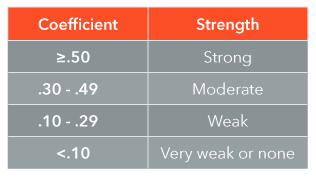Figure 14.2: Strength guidelines for correlation coefficients for the social sciences​​

### Examples: Strength of Correlations

The life insurance industry is based on statistics; in particular, insurers are in the business of predicting the likelihood that you or I or anyone else is going to die, or perhaps more accurately, when and why that will happen. These predictions involve a field known as "actuarial science," and a working understanding of them is one way in which social science students might apply some of their knowledge in a future career. We know, for example, that the more cigarettes that a person smokes, the higher their risk of developing cancer or heart disease (and the lower their life expectancy). The question is, how much higher is the risk? The stronger the pattern or association, the larger the correlation coefficient.

A person’s background can also be related to their likelihood of smoking as well as dying from smoke-related illness. Social epidemiology is a field which focuses upon the links between socio-economic characteristics and the occurrence of health and disease. Imagine that you are a social epidemiologist who wants to know more about the associations among social class, smoking, and mortality (i.e., death).

Example 1: Smoking and Mortality Rates

You have collected many years’ worth of longitudinal, national-level data on the percentage of the population that smokes at least five cigarettes per day (assuming that their habit remains fairly stable over a period of time) and their population’s mortality rate from heart disease. For example, the rate of smoking could be 1%, 10%, 25%, or more. The mortality rate is typically measured as the number of deaths per 100,000 people, such as 35, 45, and so on. A correlation coefficient of .51 would indicate that there is a strong relationship between smoking rates and mortality rates, based on population-level data. Does this establish causality? No. Does it indicate that a relationship somehow exists? Yes.

Example 2: Socioeconomic Status (SES) and Smoking

Suppose that you collected individual-level data via a cross-sectional survey (i.e., data are collected one time only, i.e., at one point in time rather than longitudinally, over time). You ask your respondents the number of cigarettes they currently smoke per day (e.g. 0, 1, 2, and so on) as well as their income. Hypothesizing that people of lower socioeconomic status smoke more cigarettes than those of higher status, you find a moderate correlation of -.30 between income and amount smoked (we will get to what the "-" sign means in the following section—it may not be what you think). Again, does this establish causality? No. Does it indicate that a relationship somehow exists? Yes.

14.03

There is no such thing as a zero correlation.

A

True

B

False

14.04

A correlation coefficient distinguishes between independent and dependent variables.

A

True

B

False

14.05

Match the following correlation coefficients with their respective interpretations of strength, according to “Cohen’s table of effect size magnitudes”.

Premise
Response
1

.22

A

Weak

2

.05

B

Very weak

3

.41

C

Strong

4

.85

D

Moderate

## Direction of a Correlation

So far, we have looked at the extent to which two variables co-vary (the strength of a correlation), but not the way that they co-vary (the direction of a correlation.) When two variables tend to increase or decrease together, their association has a positive direction. When they tend to vary in opposite directions – as one increases, the other one decreases – the relationship is a negative one (also known as “inverse”).

You can therefore have relatively strong positive or negative relationships, as well as relatively weak ones. A correlation coefficient of -.35 is equally as strong as a correlation coefficient of +.35; they differ only in terms of their direction. Think of them in terms of being on a continuum, as shown here in Figure 14.3:

There is a crucial point to emphasize here, as students often confuse it: Negative correlations can be equally as strong as positive ones. Strength and direction are two different things. The word "negative" may sound like "less," as in “my bank account balance is in the negative,” or "the temperature is -10 degrees." But in terms of correlation, the word “negative” really means "to go apart" (or "to go in the opposite direction"). Coefficients can move apart or together with equal amounts of strength, so that -1.0 is equally as strong as +1.0, and so on. The following section illustrates the meaning of positive and negative directions in greater detail.

### Common Confusion about Positive vs. Negative Direction

It can be easy to get confused by the word "negative," because the word connotes a downward tendency. If x decreases and so does y, you might be tempted to conclude that the correlation is negative because both variables go downward together. Remember, though, that to be negative means to go apart. You might remember this fact from grade school science class: if you took magnets and found that North and South poles would attract or stick together, while same poles would repel one another. The same principle applies with respect to the direction of relationships. In one case they go together (positive correlation), while in the other case they move apart (negative correlation).

If you are trying to interpret a correlation coefficient or formulate a directional hypothesis, one helpful approach is to draw arrows to represent your two variables. Let us suppose that you obtain a correlation coefficient of +.45 between two variables. Try drawing arrows to indicate the direction of the relationship:

Because the arrows go in the same direction, regardless of whether or not the arrows both point up or down, they depict a positive relationship. The higher the value on x, the higher the value on y, and vice versa. If you were instead looking at a negative correlation coefficient of -.25, that means that you should draw the arrows in opposite directions from one another. As x increases, y decreases, and vice versa.

Drawing arrows every time you interpret a correlation can help you make sense of the data. Formulating hypotheses as well as making statements about the direction of observed relationships is a persistent challenge for many students. The use of simple steps like drawing arrows can greatly reduce confusion about the logic of correlations.

The following video from the British Psychological Society illustrates the concept of correlation in an innovative visual manner, through dance. Notice its emphasis on the way that the dancers influence one another, so that as one changes, the others change as well. Both the strength and the direction of correlations are emphasized by the dancers’ movements.

Example of Positive Correlation

Many things affect how long any of us will live, including our genetic makeup as well as lifestyle behaviors such as smoking and diet. One factor that you might not have thought about is intelligence. Social epidemiologists are among the researchers who have discovered that IQ is positively associated with life expectancy (e.g. Whalley and Deary, 2001). In other words, the higher a person’s childhood IQ, the longer we expect them to live. Interest in this subject goes as far back as 1932, when during one specific day the government of Scotland gave the IQ test to nearly all 11-year-old school children. As many of these children as possible were located at age 76 to see how many were still alive; it turned out that those with higher childhood IQs had a 21% greater chance of being alive by that age.

Various studies since that time have found that IQ has a positive correlation with longevity, even after socioeconomic factors are taken into account. If we were to test this hypothesis ourselves, we would expect there to be a positive correlation between IQ and life expectancy; the higher one’s IQ, the older we expect them to live. For example, if we found a correlation coefficient of +.35, we would say that there was a moderate positive relationship between IQ and life expectancy.Figure 14.6: Direction of the correlation between IQ and life expectancy​​

There may be several explanations for this correlation, such as genetic factors that give an advantage to those with higher IQ. We do know, at least, that smarter people have an advantage, independent of their social class.

Example of a Negative Correlation

Do you often feel sleep-deprived? If so, you are not alone. Statistics Canada’s annual General Social Survey asked respondents about the ways in which they used their time, including how many hours they worked and also how many hours of sleep that they usually got, within an average 24 hour period. One particularly interesting category of people was those with at least one child under age 5. Among those respondents, there was a Pearson’s r of -.43 between the number of paid hours that they worked and the amount of sleep that they got. This is a moderate negative correlation. The more paid work that parents do, the less sleep that they get. This correlation is true of the general population but it is stronger for those people with young children, ostensibly because they have so many additional childcare duties once they are at home.Figure 14.7: Direction of the correlation between work hours and sleep​​

Among the whole sample, including everyone regardless of whether or not they had any children, the strength of the correlation was -.30, which was substantially lower in strength than among those with young children (-.43). Nevertheless, it was the same direction, in that increased work hours was associated with getting fewer hours of sleep.

14.06

Sort the following correlation coefficients in order from weakest to strongest (with the weakest on top and the strongest at the bottom).

A

-.04

B

-.99

C

+.40

D

-.12

E

0

F

-.72

G

+.01

H

+.05

14.07

Your survey results showed that there was a correlation of -.24 between employment income and number of absences from work per year. Which one(s) of the following sets of arrows depicts that relationship?14.08

Your survey results showed that there was a correlation of +.36 between number of alcoholic drinks consumed per day and number of absences from work per year. Which one(s) of the following sets of arrows depicts that relationship?## Visualizing the Correlation with a Scatterplot

Before we actually calculate correlation coefficients, we produce a scatterplot in order to get an initial sense of what the association, if any, looks like. Scatterplots (also known as scattergrams or scattergraphs) plot interval or ratio level data onto a graph in a case-by-case manner. In doing so, they give us an approximate idea of the association’s strength and direction. They also indicate whether or not the association is linear, which refers to our ability to draw a straight line through the data points (which will be illustrated in detail below). Examining scatterplots in this manner is known as visual estimation or, more informally, “eyeballing” the association.

Scatterplots are a powerful means of visualizing associations between variables so that we can understand them better, as shown in the following video. In this video, statistician Dr. Hans Rosling plots over 120,000 numbers to illustrate the correlation between per capita income and life expectancy throughout the globe over the past two centuries: 200 countries and 200 years!

In order to learn how to use scatterplots, we need to understand how they are constructed. Next, let’s examine how researchers like Dr. Rosling construct a scatterplot, although in our case we will start with just 6 data points.

### Constructing a Scatterplot

The following scatterplot (Figure 14.8) depicts the hypothetical relationship between two variables.

The scatterplot consists of two axes. Conventionally, the horizontal axis represents the predictor variable (x), while the vertical axis stands for the criterion variable (y). For those of us who have difficulty remembering which axis is which, one technique is to remember y as the letter that “stands straight up vertically with outstretched arms." It may sound silly, but it works! The points on the scatterplot are the intersection of x and y values for each specific case. We can see that the first case has a value of 0 on the predictor variable and a value of 18 on the criterion variable. The uppermost right case has a value of 18 and 101.

### Interpreting the Scatterplot

Notice that the dots in the preceding figure do not form a perfectly straight line. If they did, the relationship would have a strength of 1; however, in real life, relationships are rarely perfect. Figure 14.9 illustrates six common patterns seen in scatterplots, including varying degrees of strength.

Figure 14.9: Scatterplots illustrating common types of associations

Looking at the data points (or "dots") in the scatterplots, we can draw an imaginary line through them to get a sense of their strength and direction. When the dots point from the bottom left to the top right of the graph, it means that as x values increase, so do y values, and therefore the direction of the relationship is positive. When the data points go from the top left to the bottom right, as x values increase the values of y decrease (and vice versa), indicating that the relationship is negative. The more closely they hug that imaginary line, the stronger the relationship. When the dots are scattered randomly, there is no association, and a particular value on x does not tend to have a particular value on y. In that case, we would likely have a correlation coefficient of 0.

We might also find that a correlation coefficient = 0 in the case of the curvilinear scatterplot (which can include shapes such as U, reverse U, or a horizontal s). This is particularly the case if we are calculating Pearson’s r, which is based on a formula that assumes the association is linear. An example of a curvilinear association might be age and income. As young adults, with little education or work experience, our income tends to be quite low. As we get older and rise through the occupational ranks, our income tends to increase; however, after retirement income tends to decrease once again. To understand linearity further, let’s try adding a line of best fit to a scatterplot.

### Adding a Line of Best Fit

We can increase the interpretability of our scatterplots by adding a Line of Best Fit. A precise calculation of the best fit line will be shown in a later section. For the moment, we are only doing visual estimation (or “eyeballing”) in order to get a better picture of the form and strength of the association. Given that situation, generally speaking, our best fit line is a straight line drawn through the center of the data points that best expresses the association between the two variables. This line depicts the form of the relationship, in the sense that a straight line indicates whether or not the association is linear. If a straight line cannot be drawn through the data points, there may be no relationship at all, as shown in the right column of Figure 14.9.

On the other hand, a relationship might be a non-linear one. We always run a scatterplot before doing correlation (as well as linear regression), because the type of correlation coefficient that we should calculate depends on whether or not the association itself is linear. The following scatterplot (Figure 14.10) illustrates our best fit line for the scatterplot that we showed you earlier (Figure 14.8). Notice that we are able to draw a straight line through the data points, indicating that the association is linear. Some of the data points may touch the best fit line, but many will not.

The best fit line indicates not just linearity, but also direction, and a ballpark idea of its strength. The more closely the data points “hug” the best fit line, the stronger the association. In a subsequent section on regression, we will demonstrate a more precise method of calculating the best fit line. For now, it is enough that we are able to draw an approximate best fit line in order to "eyeball" the association and determine whether or not it is linear.

Adjust the correlation settings using the slider in the demonstration below to see the effect on the scatterplot.This Content is Locked
Only a limited preview of this text is available. You'll need to sign up to Top Hat, and be a verified professor to have full access to view and teach with the content.

### Beware of Outliers

As is the case with means and standard deviations, correlation coefficients are sensitive to outliers. You will recall from earlier chapters that outliers are extreme scores on a variable that can potentially distort your statistics so that they appear to be much higher or lower than they would otherwise be. Scatterplots are a very useful means of spotting outliers in your data, particularly when they represent cases with unusual scores between two variables.

For example, consider SAT scores (based on the older scoring system, before it was changed in the spring of 2016). Out of the approximately 1.7 million Americans who write the SAT every year, normally only a few hundred of them (about 0.02%) will achieve a perfect score of 2,400 points; even Bill Gates was 10 points shy of that achievement (The Princeton Review, 2015). Certainly, extreme values on x and/or y could distort a scatterplot’s line of best fit. The national average SAT score in 2015 was 1,490 (The College Board, 2015), although averages vary by college or university and a score of 1,700 might be average at one institution but above average at another.

Suppose that we used administrative data for 10 randomly drawn freshman students at a medium-sized college where the minimum requirement for admission is a score of 1200. We produced a scatterplot of the association between SAT scores and first-year GPA (Figure 14.11). Notice that as SAT scores rise, so do first-year GPA scores. However, there is one notable exception to this pattern: a student who had a somewhat low SAT for that institution (1400) but earned a very high GPA of 3.9. This individual is an outlier in the sense of having a relatively low SAT score but a relatively high GPA.

Outliers such as these can have a considerable impact on correlation coefficients, particularly if sample sizes are small. If we retain this particular case, we would have a Pearson correlation coefficient of .78, but if we remove it, our coefficient would increase to .97. Correlation coefficients between SATs and GPA are not usually that strong in the population, as we will touch upon again later, but for the sake of example, you can see that outliers can have a strong impact on our results. Careful examination of scatterplots can help you to spot outliers, and if necessary, you might also try calculating your coefficients with and without them to get a sense of how much they might distort your coefficients. There may also be strong theoretical reasons for keeping an outlier in the analysis, an example of which you will see later on in this chapter.

### Examples of Using a Scatterplot

A great deal of media attention and medical research revolves around the issues of excess weight and obesity. These issues are of interest to social scientists for a variety of reasons, such as socioeconomic disparities in health and illness, the role of the food industry in marketing junk food to children, and the role of governments in promoting healthy lifestyles and reducing the burden of obesity on the health care system. Social epidemiologists study ways that health and illness are distributed according to factors such as social class as well as the social determinants of health, including different lifestyle behaviors such as smoking among varying socio-demographic groups.

According to the Mayo Clinic, in order to lose one pound of fat, a person must burn 3,500 more calories than they consume (Mayo Clinic, 2016). A primary way to achieve this goal is to reduce the amount of calories consumed. With that in mind, suppose that a hypothetical sample of ten males were given a food diary to track their daily food consumption as well as the amount that they exercised, both over a three-month period. Their food consumption was converted into average number of calories consumed per day (x1), and the amount of exercise that they got was similarly averaged into the number of minutes per day (x2). The researchers also asked the subjects to track their weight during the three-month observation period. The researchers calculated subjects’ amount of weight loss over the observation period (y) by subtracting their weight at the end of the observation period from their baseline weight. The results are shown in Figure 14.12. Below, using scatterplots, we will examine the relationships between weight loss and caloric intake as well as exercise.Figure 14.12: Average daily caloric intake and exercise, and weight loss at the end of a three-month period, for a sample of men (n=10) ​

Example 1: Scatterplot for Calorie Intake and Weight Loss

With that in mind, the following scatterplot depicts the relationship between caloric intake and weight loss for our hypothetical sample of men.

To help you interpret the figures you see in Figure 14.13, look at the data point in the top left of the scatterplot. That subject consumed only 1,200 calories per day and lost 13 pounds over the 3-month period, which is not surprising given that he would have needed to consume twice that many in order to maintain his previous weight. The next two individuals consumed about 1,300 calories and lost a bit less weight, 10 and 11 pounds respectively. The individual who consumed 1,800 calories lost just five pounds.

This scatterplot depicts a negative correlation, in that the more calories they consumed (↑), the less weight the men lost in general (↓). As you can see, if you try our little trick of drawing arrows to depict the direction of the correlation, their logic can become clearer to you. In the next section, we will calculate precisely how strong that association is via Pearson’s r.

Example 2: Scatterplot for Exercise and Weight Loss

The other variable that is usually touted as a contributor to weight loss is exercise. The following scatterplot depicts the relationship between exercise (average minutes per day) and weight loss for our hypothetical sample of ten men.

In order to interpret the scatterplot correctly, examine the first data point shown in Figure 14.14. That subject exercised for an average of 7 minutes per day and lost a total of 5 pounds during the study, whilst the subject on the extreme right exercised for 15 minutes daily and lost 11 pounds. Looking at the data points overall, you will notice a different pattern than in the previous figure. This scatterplot depicts a positive correlation in that the more exercise they did, the more weight they lost in general. If you try our little trick of drawing arrows to depict the direction of the correlation, you will see that when one of them points up (or down), so does the other one.

According to this scatterplot, exercise appears to have a positive association with weight loss. However, you might notice something else: the dots are more spread apart from the best fit line than was the case with caloric intake. These results suggest that the relationship between exercise and weight loss in our hypothetical sample is not only the opposite direction than is the case for caloric intake, but it is also relatively weaker. Precisely how much weaker the relationship is will be explored in the next section.

14.09

Click on the x-axis in the following scatter plot: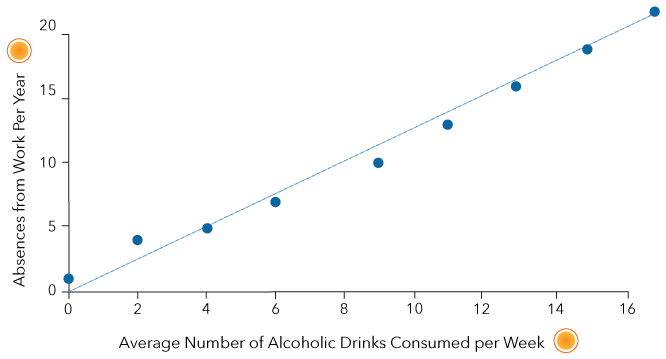14.10

The following scatterplot depicts the association between the number of hours spent watching non-educational television programs and scores on tests of reading ability among a sample of 7-year-old children (n=15). Sarah watched an average of 2 hours per day and scored 85 points (out of a possible 100 points). Click on the data point that stands for Sarah’s results.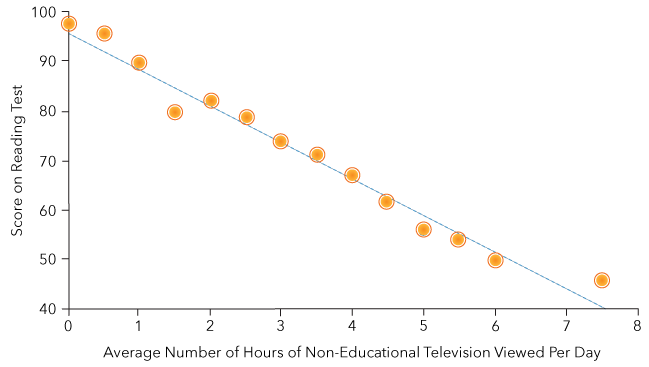## Calculating Pearson’s r

A correlation coefficient summarizes the picture portrayed by your scatterplot with a single number that represents both the strength and the direction of the relationship. If a scatterplot illustrates a ballpark estimate of the strength of an association, Pearson’s r describes its strength more precisely. Scatterplots serve another purpose in that they help tell us whether or not calculating Pearson’s r is in fact appropriate. Therefore, before we calculate Pearson’s r, we need to determine whether or not our data pass a "test" of sorts, i.e., whether or not they meet certain requirements.

### Requirements for Pearson correlation

Here are the requirements, or assumptions, for calculating Pearson’s r:

• Random sampling
• Continuous interval or ratio level data
• Normally distributed variables
• An absence of significant outliers
• A linear association between the variables

To ensure the validity of our results, we must be sure that the data come from a random sample; otherwise, bias may contaminate the results. In addition, we need to ensure that both of our variables are at least at an interval level of measurement. Think of it this way: If you were trying to draw a scatterplot of the relationship between Race and Salary, it would be impossible to determine a best fit line because it would be nonsensical to suggest, for example, that salary increases as race increases. Race cannot increase or decrease because it is nominal (categorical). In addition, the variables should be normally distributed, with an absence of significant outliers. As you saw in a preceding section, Pearson’s r is sensitive to outliers, and they can lead to misleading results. Finally, the association should be linear, which you can determine by creating a scatterplot prior to calculating your coefficient. Pearson’s r cannot detect non-linear associations, which can be quantified by other measures such as Spearman’s rank correlation, illustrated later on in this chapter.

### Formula and Steps for Calculating Pearson’s r

The equation for Pearson’s r is as follows:

At first glance, this equation might appear very daunting in its complexity. However, you will see below that it actually involves just a few calculation steps:

These steps can be aided with the use of a table in order to keep all of your calculations organized. Below are detailed examples of how to do so.

Try your hand at estimating the correlation represented by the scatterplots in the demonstration below.This Content is Locked
Only a limited preview of this text is available. You'll need to sign up to Top Hat, and be a verified professor to have full access to view and teach with the content.

Example: Calories and Weight Loss

Let us return to the example of the amount of calories consumed and the amount of weight loss by individuals in our hypothetical sample of ten men.

Note: For the sake of simplicity, calories are expressed in units of 100. This sort of conversion is often done to simplify the presentation of data and has no effect on the final result when calculating a statistic like r.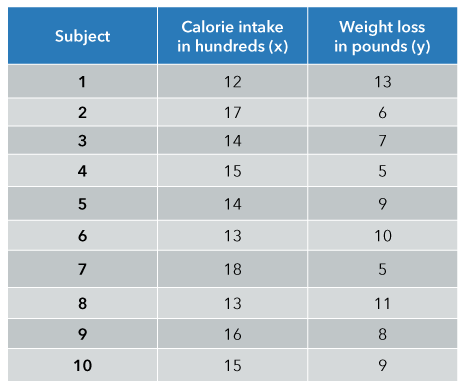Figure 14.16: Average daily caloric intake (converted to hundreds) and weight loss at the end of a three-month period, for a sample of men (n=10)​​.​​​

Step 1: Calculate xy, x², and y².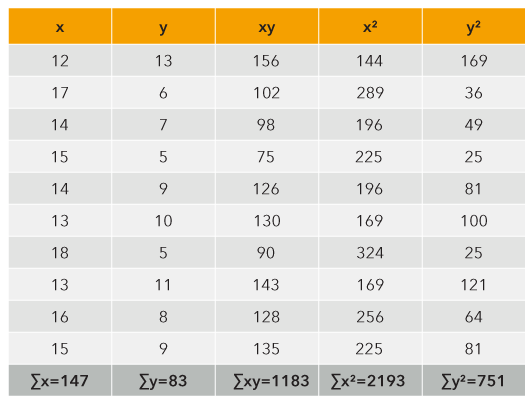Figure 14.17: Calculating Pearson’s r between caloric intake and weight loss​​.​

Step 2: Calculate the correlation coefficient (r). Interpret the results.

Step 3: Interpret the results.

In order to interpret the strength of this correlation, refer back to the guidelines for interpreting strength (Figure 14.2), which indicate that we can consider it to be “strong.” We could construct an interpretive statement along the following lines, which should include both the strength and the direction of the coefficient: “The Pearson correlation coefficient (r) of -.83 tells us that there is a strong negative relationship between caloric intake and weight loss in men.”

Example: Exercise and Weight Loss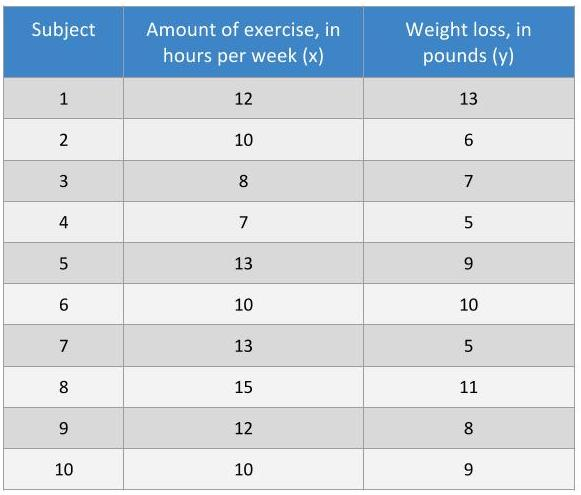Figure 14.18: Average amount of exercise (in hours per week) and weight loss (in pounds) at the end of a three-month period, for a sample of men (n=10).​

Step 1: Calculate xy, x², and y².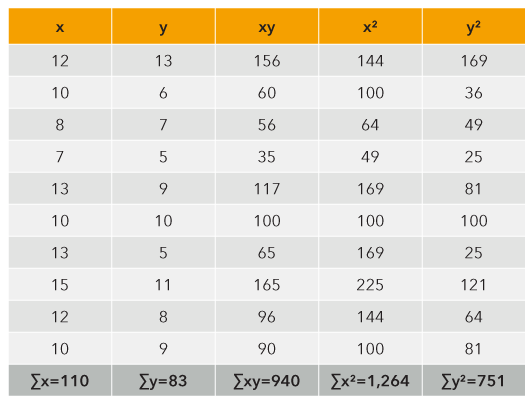Figure 14.19: Calculating Pearson’s r between amount of exercise and weight loss

Step 2: Calculate the correlation coefficient (r).

Step 3: Interpret the results.

In order to interpret the strength of this correlation, refer back to the guidelines for interpreting strength (Figure 14.2), which suggests that we can consider it to be “moderate.” We can put these results into an interpretive statement, such as: “The Pearson correlation coefficient (r) of +.47 tells us that there is a moderate positive relationship between exercise and weight loss in men.”

### Correlation Coefficients as a Means of Comparing the Relative Strengths of Associations

By now, you have likely noticed something else about the relative strengths of the associations between weight and caloric intake versus exercise: they differ quite a lot. An advantage of Pearson coefficients is that because they possess a common metric (standard deviation units), they allow us to compare the strengths of relationships with one another, much as we use standard scores like z-statistics to compare scores across distributions. In our example, correlation between caloric intake and weight loss is strongly negative (r = -.83), while the correlation between exercise and weight loss in the example above is comparatively weaker (r = +.47). While these are hypothetical results, they illustrate an established finding in the medical literature on weight loss, and one that might be disappointing to many of us: What we put (or do not put) in our mouths will ultimately have a much stronger impact on our weight than the amount of exercise that we get.

A recent article in the New York Times (Carroll, 2015) caused a bit of a media sensation when it highlighted this fact, noting that the public is led to believe otherwise through sweat-to-lose approaches seen in popular television shows like The Biggest Loser and in advertising from a massive fitness industry. According to what are known as “meta-analyses” of existing studies, the correlation between exercise and weight loss is relatively weak. Dr. Gary Wenk, Professor of Psychology and Neuroscience at Ohio State University and Medical Center, explains how the amount of food eaten impacts longevity, with emphasis upon the relatively weak utility of exercise to lose weight (and in turn, to live longer):

That said, there is a variety of variables that have a combined relationship with weight loss, including genetic factors. But we can at least say that certain variables have a stronger association with weight loss than other variables do.

14.11

One of the requirements for Pearson’s r is that a $\_\_\_\_\_\_\_\_\_$ sampling method should be used.

14.12

Calculate Pearson’s r for the following data. Note that we conventionally calculate Pearson’s r to two decimal places and include a sign (+ or -) to indicate its direction.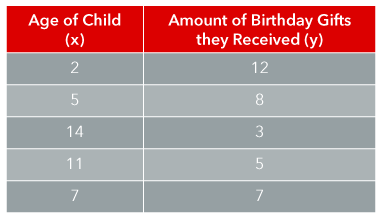## The Coefficient of Determination

Correlation coefficients provide us with a very precise idea of the extent to which two variables are associated. But what we consider “strong,” “weak,” and so on is somewhat subjective. Some social scientists (and statistics textbook authors) might deem a coefficient of .45 to be fairly low in strength, while others might consider that to be notably strong, depending on their discipline and the context of their research. To some extent, we can get a more precise benchmark of the strength of a Pearson correlation coefficient by squaring it. Therefore if r = .45, r² = .20.

The coefficient of determination (r²) refers to the amount of variation in one variable that is explained or accounted for by the variation in another variable. Therefore, r² is also known as a measure of explained variation. It can be viewed as the amount of variation in y that is attributable to the variation in x, or the amount of variation in x that is attributable to the variation in y. If r² =.20, it means that 20% of the variation in x is attributable to the variation in y, and vice versa. The remaining percentage (i.e., 100 - r²) is the unexplained variation. It means that the variation in y is accounted for by some other variable(s) other than x.

The coefficient of determination can be viewed not only as a measure of explained variation, but also as a measure of the strength of the association. What might appear at first glance to be a relatively strong association has a way of diminishing in magnitude when r is squared. For example:

If r=.70, r² = .49 (or 49%)

If r =.50, r² = .25 (or 25%)

If r = .25, r² = .06 (or 6%)

While r = .50 appears to be twice as strong as r = .25, it in fact explains four times as much variation (25% versus 6%). Much of the interpretive power of r therefore lies in the coefficient of determination. We should always bear in mind that if the value of r is lower than .31, the variation in x will explain less than 10% of the variation in y (and vice versa).

Examples

The correlation between caloric intake and weight loss in our previous example (using hypothetical data) was -.83. Therefore, the variation in caloric intake explained 69% of the variation in weight loss, as (.83)² =.69. The remaining 31% remains unexplained. For example, weight loss can also be associated with other factors such as exercise, genetic makeup, and height.

The correlation between exercise and weight loss was much lower (r=+.47). The coefficient of determination tells us that just 22% of the variation in weight loss is explained by the variation in amount of exercise, and vice versa. We arrived at this figure by calculating (.47)²=.22. The remaining 78% remains unexplained and, similar to the above example, it might be accounted for by factors such as caloric intake, genetic makeup, and height.

## Rank Correlation

There are many situations in which our variables are associated, and for which Pearson’s r is not appropriate. This is especially the case when your variables are not at least at an interval level of measurement, or if their association is non-linear. In cases like these, rank correlation can often be used in place of Pearson’s r

Like other correlation coefficients, rank correlations vary between 0 and ±1, and can be positive or negative. There are different types of rank correlation commonly used in social research, including Gamma, Kendall’s tau b, and, perhaps most commonly, Spearman’s rho (rs). Since it is beyond the scope of this text to cover all of them, we will focus on Spearman's rank correlation.

Spearman rank correlation is often described as a form of Pearson correlation in that its calculation is similar, but instead of using raw data values it uses ranked values. The requirements for using Spearman’s rho differ from Pearson’s r in that the variables do not need to be interval/ratio level, nor do they need to be linearly associated. However, they do need to meet the following requirements:

• Random sampling
• Both variables must be at least ordinal
• The variables must increase monotonically with one another

Monotonicity refers to whether or not one set of scores tends to increase or decrease alongside another set. Linear associations (which are a requirement for the calculation of Pearson’s r) are monotonic, but they also form a straight line (are linear). As you can see in the middle scatterplot of Figure 14.20, an association can be non-linear and monotonic, in which case the scatterplot shows an increasing or decreasing association without forming a straight line. In those cases, Spearman's rank correlation is a common alternative to Pearson’s r. However, to be monotonic, scores cannot increase together and then decrease; those are non-monotonic associations, like the one shown by the scatterplot at the far right. In those cases, neither Pearson’s r nor Spearman’s rho are appropriate to use. (There are other ways of quantifying those types of relationships, such as Cramer’s V, but they are beyond the scope of this text.)

Example: The Halo Effect (Rank Correlation for Ordinal Variables)

Take a look at this article from CNN. Does the individual in the video look familiar?

If you spend any time on social media, you will probably recognize the man in the video as Jeremy Meeks. His mug shot was released by the Sacramento Police Department in 2015 and went viral within hours of being posted on their Facebook site, leading Meeks to be dubbed “the hot convict” (The Independent, 2016). Despite the fact that he was a convicted felon with a violent criminal and gang history, women from all over the world publicly commented on how much they were enamored of Meeks.

Writing for the magazine Psychology Today, veteran lawyer Wendy L. Patrick (2014) points out that good-looking people like Meeks often have a lot of “jury appeal,” and in some circumstances, this might work to their advantage in court. Patrick was referring to a well-established psychological concept known as the “halo effect.” It is a type of cognitive bias in which people tend to equate looking good with being good (including being honest, trustworthy, and intelligent). In fact, this concept is nothing new; it originated in rank correlational analyses performed nearly 100 years ago.

In 1920, psychologist Edward Thorndike (1920) conducted a study in which he asked members of the army to rank their fellow members on characteristics such as intelligence, physical qualities (include voice, bearing, neatness, and endurance), leadership potential, and overall character. They were asked to create a set of ranks for members of the same rank, and then did the same for subordinates. Each member was asked to create a scale by naming the fellow member whom they felt had the highest rank at the top, the lowest name at the bottom, and one in the middle, followed by one in between the bottom and middle and between the middle and top, for a total of five ranks. They created a scale for physical qualities, then repeated the process for intelligence, and so on.

Members were instructed to rank each of the variables independently of one another, meaning that in ranking fellow members in terms of intelligence, for example, they should not take into consideration who received which ranks for physical qualities and so on. However, that is exactly what happened. For example, among officers of the same army rank as their own, there were strong correlations between physique and the other variables, including:

• Intelligence (.51)
• Overall character (.54)

When these members ranked their subordinates, the overall pattern between the variables was the same.

Clearly, there are benefits to being good-looking that go beyond the dating realm. For example, various studies testing the Halo Effect have discovered things like:

• A man of 6’5” in height can expect to earn \$5,525 more per year than a man of 5’5” in height (Judge and Cable, 2004).
• Students will give higher ratings of a professor’s appearance, mannerisms, and foreign accent if the professor initially comes across as warm and friendly vs. cold—meaning that first impressions do indeed count (Nisbett and Wilson, 1977).

Do you still find it hard to believe that people could be so prone to judging your character by things such as your appearance? Check out this field experiment from the UK; it will convince you that Thorndike was right.

In the next section of this chapter, we will calculate a rank correlation that further illustrates how the Halo Effect works mathematically. This example has illustrated why ordinal variables with ranked scores are appropriate for rank correlation. The following example works a bit differently. This time, the data are interval/ratio, and they illustrate the other main reason for calculating Spearman’s rho rather than Pearson’s r: situations in which an association is curvilinear and monotonic.

Example: Literacy and Fertility in India (Rank Correlation for Curvilinear Associations)

If you have ever taken a course in demography, you may have heard of a rather unique example of how an otherwise poor region of the world can undergo a “demographic transition” to the point at which their life expectancy is on par with wealthy countries like the U.S., Canada, and Norway. Kerala, India became well-known as the first region of India to claim nearly 100% literacy, and they were pioneers in advancing women’s literacy in particular. Kerala’s campaign of social reform dates back to the late nineteenth century and early twentieth century, and it now has the highest Human Development Index (HDI) value and lowest population growth rate in India. Note in the following figure that Kerala and the majority of the surrounding states have low fertility (1.6 to 1.8 children born during a woman’s lifetime), which is in fact below replacement level compared with the states to their north, which are high (2.3-3.4 children per woman).

Demographers have long maintained that female literacy and fertility are negatively associated. The greater a woman’s education, the fewer children she is likely to have, in large measure because she will have greater participation in the economy and hence delayed childbearing. In order to examine the Indian case, we should start by producing a scatterplot to get a visual estimation of the association between literacy and fertility, and determine what sort of correlation coefficient we should calculate. We know that literacy and fertility are at interval/ratio levels of measurement, so we might just assume that we should go ahead and calculate Pearson’s r. Look at Figure 14.22 below; can you see why Spearman’s rho is a better option?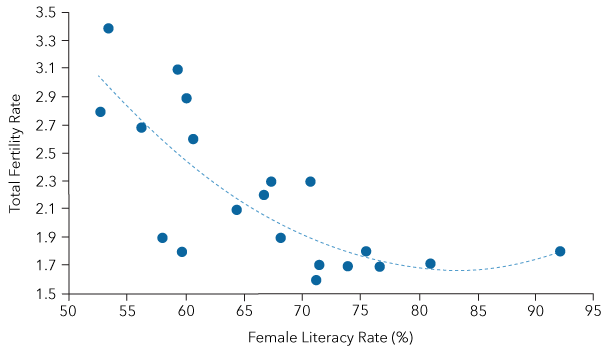Figure 14.22: Scatterplot of female literacy and fertility rates among major Indian states, 2014​​

Note that female literacy and fertility display a monotonic pattern. However, by the time a state reaches about 70% literacy, we start to see diminishing returns, and fertility rates hover around 1.7 children per woman. That is why the best fit line starts to plateau at that point, making the association curvilinear. (Kerala is moderately an outlier on literacy, but even if we were to remove it from the scatterplot, the pattern would still be curvilinear. Moreover, Kerala is important to retain for theoretical reasons illustrated above.) We could, in this case, simply collapse each variable into a few categories, such as low, medium and high fertility, and do the same for literacy, and place them into a contingency table. However, that would mean a substantial loss of information in terms of the finer distinctions between our cases. At the same time, we cannot calculate Pearson’s r because it would require a linear association. In such cases, ranking the cases and calculating Spearman’s rho is our best option; we will do so in the following section.

14.13

Among the following three scatterplots, in which case would Spearman’s rho be most appropriate to calculate?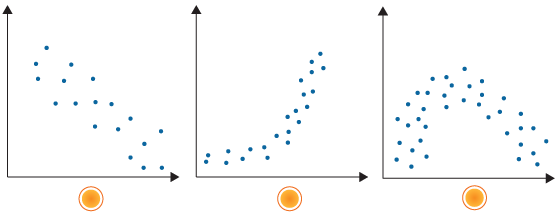14.14

When a set of x and y values increase or decrease together but never increase and subsequently decrease together, we say that they are $\_\_\_\_\_\_\_\_\_\_\_\_.$

### Calculating Spearman’s rho (rs)

In order to calculate Spearman’s rho (rs), we use the following formula:

Where

d = the difference between the ranked values on each variable

d² = the difference squared

n = the number of cases of paired values

∑d² = the sum of the squared differences between rankings on each variable

We can walk through this equation using the following steps.

The clearest way of calculating these steps is through the use of a table, as you will see in the following examples.

Example: The Halo Effect

Suppose that we have asked a sample of 15 women to view photos of eight men in their thirties. They were asked to rate each of the men on a scale of 1-10 in terms of attractiveness. They were also asked to rate each of the men from 1-10 in terms of how intelligent they thought the men appeared to be. The men’s total ratings scores were then calculated by adding together all of the women’s ratings, so that a perfect score would be 150 (i.e., rated 10/10 by all of the women). Researchers then ranked the men according to their total scores on attractiveness and intelligence, with lower numbers reflecting higher ranks (akin to 1st place, 2nd place, and so on). The results are shown in the first three columns below (note that for clarity, the table illustrates only the ranks rather than the original scores):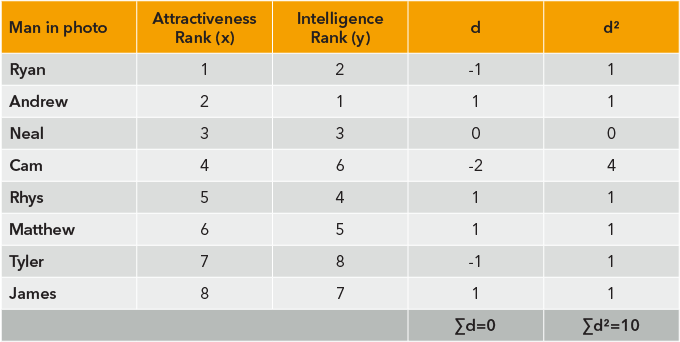Figure 14.24: Hypothetical rankings of the attractiveness and intelligence of eight men​

Because the women’s ratings were pooled and the eight men ranked, we consider the men to be our cases (n=8). You will notice that we have completed Step 2 in the fourth column, “d” (calculate the difference between ranked values on x and y), as well as squaring those differences, or “d²” (Step 3) in the last column and summing them (Step 4). Next, we can calculate Spearman’s rho using the formula (Steps 5 and 6):

Our hypothetical results indicate that there is a strong positive correlation of +.88 between attractiveness and perceived intelligence. They would support the notion of a halo effect, in that higher rankings of attractiveness are associated with other positive personal qualities.

Example: Literacy and Fertility in India

In this example, we will use real-life data to test the argument that the potential for human development is greater when a society fosters education for women. Literacy and education are considered to be key indicators of human development. Reintroducing the data that you saw in a previous section on scatterplots (Figure 14.21), the following table illustrates raw data values for fertility (x) and literacy (y) among 20 states in India. We have placed y first in the table because we want to emphasize the rank of fertility as a key indicator of human development cross-nationally. In this example, we have ranked the states in terms of lowest fertility level (rank = 1) to highest fertility (rank = 20). Conversely, literacy has been ranked according to highest percentage of female literacy (rank = 1) to lowest percentage (rank = 20).Figure 14.25:​ Literacy and fertility rates and rankings among twenty Indian states​

You will notice a difference from the previous example (the Halo Effect), in that the values of x are not simply ranked as 1, 2, 3, and so on, but they can include a decimal place. The reason is that some cases in this example have duplicate or “tied” values/scores, such as the second through the fifth states, all of which have a fertility rate of 1.7. This is a very commonly encountered situation. If cases have the same score, we assign them the mean of the ranks that they would have held if they had not been tied. For example, those four cases would be divided by 4, and all of them receive the same rank: (2 + 3 + 4 + 5)/4 = 3.5.

As was the case in the previous example, the results for Steps 2 through 4 are indicated in the table. Next, we can calculate Spearman’s rho using the formula:

We can conclude from these results that there is a strong relationship between the female literacy rate and the total fertility rate in India. The higher a state’s female literacy, the fewer number of children women are likely to have during their lifetime, but only up to a certain threshold. These results suggest that India is quite heterogeneous in terms of the demographic transition. States in the South have placed greater emphasis upon women’s education, and their subsequent economic participation has led to delayed childbearing, which is considered by demographers to be a key determinant of a country’s level of human development.

14.15

The following table depicts the values of two variables for five countries. The first variable is the UN’s Human Development Index (HDI) which is an indicator of human development that takes into account things like life expectancy and income. The top five ranked countries in the world (for the year 2014) are depicted in the table. The second variable is the UN’s Gender Inequality Index (GII), which is an indicator of the level of gender disparity in a country.

If we were to rank HDI values so that the country with the highest HDI received a rank of “1”, what would be the rank for Switzerland?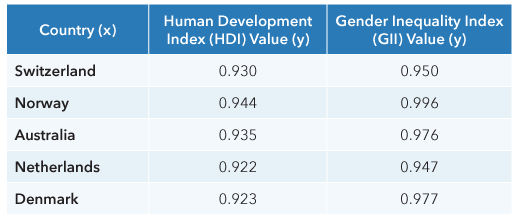14.16

The following table depicts the values of two variables for five countries. The first variable is the UN’s Human Development Index (HDI) which is an indicator of human development that takes into account things like life expectancy and income. The top five ranked countries in the world (for the year 2014) are depicted in the table. The second variable is the UN’s Gender Inequality Index (GII), which is an indicator of the level of gender disparity in a country.

What would be the HDI rank for the Netherlands?14.17

The following table depicts the values of two variables for five countries. The first variable is the UN’s Human Development Index (HDI) which is an indicator of human development that takes into account things like life expectancy and income. The top five ranked countries in the world (for the year 2014) are depicted in the table. The second variable is the UN’s Gender Inequality Index (GII), which is an indicator of the level of gender disparity in a country.

Calculate Spearman’s rho between the HDI and GII in order to test whether nations with higher levels of human development also have lower levels of gender inequality. (Hint: The country with the lowest GII score should receive a rank of “1”.) Which of the following values comes closest to the one that you calculated?A

-0.09

B

+0.44

C

+0.70

D

+0.86

## Significance of a Correlation

As you probably gathered, the concept of statistical significance does not mean whether or not a particular result is “important,” “meaningful,” “worth noting,” and so on. Those things are known as substantive significance. Rather, statistical significance refers to the probability that results as large as we observed are due to sampling error, or chance. This statement implies that there exists a degree of uncertainty that any relationships that we observe in a sample actually exist in the population from which the sample was drawn.

Pearson’s r is a sample-based estimate of the population value rho, denoted as the Greek symbol Ρ, while Spearman’s rho (rs) is likewise an estimate of the population value rho, but denoted as Ρs. The stronger the correlation and the larger the sample size, the more likely the coefficient is to be statistically significant. Even relatively weak Pearson’s r or Spearman’s rho values are likely to be significant if the sample size is reasonably large.

In order to determine the significance of our coefficient, there is, as the saying goes, “more than one way to skin a cat.” Two distributions are commonly used to test the significance of correlation coefficients covered in this chapter: the Student’s t-distribution and the F-distribution. We have chosen to use the t-distribution.

The equation for the significance of r is as follows:

Similarly, the equation for the significance of rs is as follows:

Where r (or rs) = the correlation coefficient

n = the number of cases

The steps for testing the significance of correlations are summarized in the table below.

Example: Caloric Intake and Weight Loss (Pearson’s r)

This example expands upon the previous example, in that we already calculated how strong the association is between caloric intake and weight loss, but we also need to know if it is significant or not.

Step 1: State the claim and identify the null and alternative hypotheses as H0 and H1.

Our sample findings suggest that there is a strong negative association (r = -.83) between caloric intake and weight loss. Our null hypothesis (H0) is that there is no association between caloric intake and weight loss in the population. Our research hypothesis (H1) is that there is a correlation in the population, meaning that it is different from zero. These points can be summarized as follows:

Step 2: Specify the level of significance, represented as α.

We normally set a level of significance, or alpha level, in advance. By doing so, we can establish the probability that our result is due to chance. In this case, given the fact that our sample is quite small, we will select the .05 level of significance, or p<.05.

Step 3: Identify the critical value of the test statistic (in this case, t) to indicate under what conditions the null hypothesis should be rejected or not rejected.

To obtain the critical value of t, we need to calculate the degrees of freedom (df) for Pearson’s r (n-2). Therefore, the df are: 10 – 2 = 8. Next, we look up the critical value of t in the t-distribution.

For the .05 level of significance for a two-tailed test with 8 degrees of freedom, the critical value is 2.306.

Step 4: Calculate the test statistic using data from the sample:

Step 5: Compare the calculated statistic to the critical value and decide to reject or fail to reject the null hypothesis.

Because t = 4.22 exceeds the critical value of 2.308 (p<.05), we reject the null hypothesis that there is no association between caloric intake and weight loss.

Step 6: Interpret the decision in terms of the original claim.

By rejecting the null hypothesis, we can conclude that the correlation between caloric intake and weight loss (r=-.83) is statistically significant at the .05 level. The probability that our results are due to chance is less than 5%. It should be added, however, that with such a small sample size (n=10), in order for an association to be statistically significant, it has to be very strong. In general, the stronger the association and the larger the sample size, the more likely the association is to be statistically significant. Even relatively weak correlation coefficients are likely to be significant if the sample size is relatively large because the probability of obtaining a chance result decreases.

Example: Female Literacy and Fertility in India (Spearman’s rho)

Again, we can expand upon the example in the previous section by determining whether or not the coefficient that we calculated earlier is significant or not.

Step 1: State the claim and identify the null and alternative hypotheses as H0 and H1.

Our sample findings suggest that there is a strong negative association (r = -.77) between a country’s female literacy rate and its fertility rate. Our null hypothesis (Ho) is that there is no association between a country’s female literacy rate and its fertility rate in the population. Our research hypothesis suggests that there is an association in the population, meaning that it is different from zero. These points can be summarized as follows:

Step 2: Specify the level of significance, represented as α.

We normally set a level of significance, or alpha level, in advance. By doing so, we can establish the probability that our result is due to chance. In this case, we will select the .01 level of significance, or p<.01.

Step 3: Identify the critical value of the test statistic (in this case, t) to indicate under what conditions the null hypothesis should be rejected or not rejected.

To obtain the critical value of t, we need to calculate the degrees of freedom (df) for Pearson’s r (n-2). Therefore, the df are: 20 – 2 = 18. Next, we look up the critical value of t in the t-distribution table. For the .01 level of significance for a two-tailed test with 18 degrees of freedom, the critical value is 2.878.

Step 4: Calculate the test statistic using data from the sample:

Step 5: Compare the calculated statistic to the critical value and decide to reject or fail to reject the null hypothesis.

Because t = -3.80 exceeds the critical value of -2.878 (p<.01), we reject the null hypothesis that there is no association between a country’s female literacy rate and its fertility rate in the population.

Step 6: Interpret the decision in terms of the original claim.

By rejecting the null hypothesis, we can conclude that the correlation between female literacy and fertility (r = -.77) is statistically significant at the .01 level. The probability of obtaining these results if the null hypothesis is true is less than 1 percent.

14.18

Sort the following steps for testing the significance of a correlation.

A

Specify the level of significance represented as α.

B

Interpret the decision in terms of the original claim.

C

State the claim and identify the null and alternative hypotheses as $H_0$ and $H_1.$

D

Calculate the test statistic using data from the sample.

E

Compare the calculated statistic to the critical value and decide to reject or fail to reject the null hypothesis.

F

Identify the critical value of the test statistic (in this case t) to indicate under what conditions the null hypothesis should be rejected or not rejected.

14.19

Suppose that we calculated r$_s$ = .21 (n=15). Do we reject or fail to reject the null hypothesis at p<.01?

A

Reject

B

Fail to reject

14.20

For the same data as the previous question, do we reject or fail to reject the null hypothesis at p<.05?

A

Reject

B

Fail to reject

## Correlation and Causality

One of the most familiar refrains that you will hear in any Introductory Statistics course is “correlation is not causation,” and it is one with which social scientists and other assorted statistics geeks tend to have a field day with. In fact, they have so much fun with it that there is an entire website devoted to absurd correlations. For example, some of their recent online polls, in which you can participate by logging onto their site or reading their book (Shaun Gallagher 2014), found that:

• People who have tried a fad diet in the past three years are more likely to be physically affectionate than everyone else.
• People who prefer Miss Piggy over Kermit the frog are more than twice as likely to have tattoos.
• People who think that a day out fishing on a boat is enjoyable are more likely to like new car smell.

Their point is that many things in life will be correlated, but many of those correlations are merely coincidental. As much as we humans tend to be pattern-seekers—it is a survival skill¬—sometimes these patterns really don’t mean much of anything at all, or they can be accounted for by additional variables. Because statistics like Pearson’s r don’t distinguish between independent and dependent variables, we need to use caution when interpreting them, causally speaking.

Regardless, we often make errors in causal reasoning that make us vulnerable to misunderstanding what is really going on. There are various types of errors in causal reasoning that social researchers try to avoid. Let’s examine three of the more prominent ones:

• Spuriousness
• Reverse causation
• The post-hoc fallacy

### Spuriousness

While coincidences like those illustrated above are amusing, on a more serious note, we should stress that it is vital not to “jump to conclusions” whenever we find a correlation between two variables. The consequences can be potentially dire if they lead to a failure to consider other possible explanations for the association. A spurious correlation is one in which the association is caused by a third variable that affects both of the association’s two variables simultaneously.

In this chapter’s opening example, WHO Director Dr. Margaret Chan was very careful to explain to the media that a causal relationship between the Zika virus and microcephaly had not yet been established at that point in time. Other possible causes for the association between microcephaly and Zika needed to be explored. We are reminded why it is important to exercise such caution by another historical pandemic in children: polio. Watch famed Freakonomics authors Steven Levitt and Stephen Dubner describe how early in the twentieth century, it was thought that ice cream consumption was a cause of polio in children, which we now know was a virus that caused devastating effects such as paralysis.

In the case of ice cream and polio, a confounding variable was “lurking” in the background: the season. The warmer the weather (i.e., the summer months), the more likely people were to eat ice cream, and also the more likely an outbreak of poliovirus was. Confounding variables correlate with both of the associated variables at the same time.

We try to avoid spuriousness by controlling for various potentially confounding variables; we call them control variables. “Control” means that we hold a variable (such as season) constant, i.e., not allowing it to vary. We could hold season constant by calculating the correlation between rates of ice cream consumption and rates of polio during summer months only, and re-calculating the rates for the other months of the year. The causal diagram would look like this:Figure 14.28: Diagram of spurious correlation between ice cream consumption and polio rate​.

When we control for season, we find that the association between ice cream consumption and polio disappears. Season was a confounding variable.

### Reverse Causation

There are other ways in which correlations are not causal, or at least not in the way we think they are. One type of confusion is a reversal of cause and effect. For example, a recent study by Liu et al. (2016) reported that frequent users of social media (such as Facebook) were 2.7 times more likely than other people to be depressed, even after controlling for factors like gender and income. It may be the case that these individuals are more likely to be subject to bullying, or simply realize that they are wasting a lot of time, for example. The researchers were quick to acknowledge, however, that it is hard to determine cause vs. effect based on correlational analyses and cross-sectional data. It may be the case that many people who are already more likely to be depressed tend to turn to social media to cope. But the strong correlation suggests that further studies to determine the nature of the causal relationship are highly warranted.

### The Post-Hoc Fallacy

A common error in causal thinking is “post-hoc reasoning”, which refers to the Latin phrase post hoc ergo propter hoc, meaning “after this therefore because of this” – i.e., if B followed A, then A must have caused B. (A similar but perhaps less frequently cited fallacy is cum hoc ergo propter hoc, meaning “at the same time as this therefore because of this.”) In other words, patterns of events are assumed to be relationships of cause and effect. Indeed, a necessary condition for causality itself is that the cause must precede the effect; however, it is an insufficient condition on its own. Maybe the apparent effect would have occurred anyway, or maybe the effect was caused by something other than the suggested cause.

Post-hoc reasoning is fallacious in that it leads us to neglect alternative explanations for an event. Other explanations that have been explored for microcephaly, for example, include local water contamination, malnutrition, and other viruses. This is a different issue from spuriousness in that these other variables might explain the outcome (the increase in microcephaly) but not the apparent cause (an increase in Zika virus). In the sense of ignoring other possible causal explanations for a phenomenon, however, post-hoc reasoning is also a contributor to spurious reasoning.

### However… the Stronger the Association, the More Likely that it is Causal

While correlation does not necessarily guarantee causation, on balance, it is critical to bear in mind the notion that “where there is smoke, there is fire.” Just as it can be easy to jump to erroneous conclusions about causation based on correlation alone, the corollary is that it can be easy to dismiss correlations for lack of firmly established causal evidence. The stronger the correlation, the more likely there is causation.

Claiming that something is “just a correlation” is, as epidemiologist Sir Michael Marmot states in the following video, often a “cheap shot” meant to dismiss potentially important causal explanations of a phenomenon, as was once the case between smoking and lung cancer.

Science relies on two key qualities in order to make any reliable sort of claim. The first one is empirical support, which indicates that we have gathered observable evidence using our senses. The second one is logical support, which lies in the mind of the researcher who theorizes that the theory or explanation for some event makes sense. In the video, Marmot illustrated how the use of scientific logic led to in-depth empirical investigation (and ultimately, support) of the hypothesis that smoking is a primary cause of lung cancer, even though naysayers previously argued otherwise.

But correlation alone will not usually be sufficient empirical evidence of causation. The use of control variables helped solidify the argument that smoking was indeed a cause of lung cancer, i.e., independent of variables like a person’s genetic makeup. To control for these various other factors, researchers often use a technique called regression. In the next section, we will explore simple linear regression between two variables. Although controlling for a variety of variables is beyond the scope of this textbook, learning about simple regression will help you understand the basic logic behind the more complex types of regression analyses that researchers rely on in order to establish causal relationships.

Example: Post-Hoc Reasoning

Suppose that we observed that “Immigration to California from Mexico increased. Soon afterward, the welfare rolls increased. The correlation between immigration rates and welfare rates is +.47. Therefore, the increased immigration caused the increased welfare rolls.” Immigrants may be the reason for the uptick in welfare use. But perhaps welfare rolls would have increased anyway, or perhaps the effect was caused at least in part by something other than immigration.

Example: Spuriousness

A classic little book in the area of statistical logic is Darrel Huff’s How to Lie with Statistics (1954). Huff observed that it was a statistical fact that there was a positive correlation between the salaries of ministers in Massachusetts and the price of rum in Havana, Cuba. Huff noted that concluding that salaries cause the price of rum to increase is flawed causal reasoning. What happens when we control for global inflation?

When we take into account global inflation, the association between salaries and the price of rum disappears, and r=0. Global inflation is a confounding variable that causes both salaries and the price of commodities to rise at the same time.

14.21

Which of the following scenarios illustrates a spurious correlation?

A

The more cigarettes smoked, the lower the life expectancy

B

The fewer hours studied, the higher the grade

C

The more firefighters who arrive at a fire, the greater the fire damage

14.22

You decide to ritualistically eat apple cinnamon oatmeal for breakfast on exam days because you got an “A” on your statistics exam on a day that you had consumed a large portion of it. This is an example of what sort of reasoning?

A

a priori

B

post hoc

C

intuitive

## Simple Linear Regression

Correlation and regression are similar to one another in the sense that they both describe relationships between variables. Regression is a logical extension of Pearson’s r, going a step further by allowing us to make more specific predictions about values of y when we know values of x. To do so, we will revert from z-scores back to our original raw scores.

### Reintroducing the Line of Best Fit

We previously saw how a line of best fit could be “eyeballed” to see if a scatterplot appeared to indicate a linear relationship between two variables. The line of best fit (also known as the regression line) can more accurately be specified by the linear regression equation:

Where ŷ = our predicted value of the response (or “outcome”) variable.

x = the value of the predictor variable;

a = the intercept;

b = the slope of the regression line, or its steepness.

Let’s examine each of these components in detail.

### Components of the Regression Equation

If the regression equation allows us to predict values of y when we know the value of x, then first we will need to determine the slope (b) and the intercept (a). These are actually very straightforward equations.

The slope (b) is the steepness of the best fit line; it represents the amount of change in y for every unit of change in x. In that sense, the slope is a type of descriptive statistic. To calculate the slope, we use the following equation:

The intercept (a) is the point at which the regression line crosses the y-axis when x=0. To calculate the intercept, we use the following formula:

Where y̅ = the mean of all y values in the distribution,

x̅ = the mean of all x values in the distribution, and

b = the slope, i.e., the steepness of the best fit line.

The general steps for applying the regression equation are summarized in the table below.

Experiment with the settings for sample size, slope of the regression line, and the standard deviation to see the effect on the scatterplot and the line of best fit.This Content is Locked
Only a limited preview of this text is available. You'll need to sign up to Top Hat, and be a verified professor to have full access to view and teach with the content.

Sample size is a critical measure when considering correlations. Here's a good example:

Example: Calorie Consumption and Weight Loss

We know already that there is a strong negative correlation (r= -.83) between caloric intake and weight loss in our hypothetical example. For someone who consumes just 1500 calories per day, how much weight do we expect them to lose? We already know the value of x (1,500), and want to predict the value of ŷ. To use the regression equation, we first need to determine the slope (b) and the intercept (a).

Step 1: Calculate and interpret the slope (b)

Recall that the formula for calculating the slope (b) is as follows:

The clearest way to illustrate the computation of this formula is to present its components in the form of a table (as below). It will make the formula itself much less intimidating. You will likely notice, however, that you have seen this same table before. The numerator of the formula for the slope (b) is exactly the same as the numerator of the formula for Pearson’s r because both of those formulae require that we calculate the covariation of x and y. For the sake of clarity, however, we will present these data again.Figure 14.31: Calculation of the slope (b) between calorie intake and weight loss​

Using the data in the table, we can now calculate the slope more easily since we included some of the basic calculations within the table:

Make sure to understand how to interpret b. It means that for each extra 100 calories consumed (since we measured intake by hundreds of calories), amount of weight loss will decrease by 1.16 pounds. While that sounds like a relatively small amount, when you consider the number of calories people consume per day, those quantities add up significantly.

Step 2: Calculate and interpret the intercept (a).

Next, using data from that same table, we need to calculate mean caloric intake as well as mean weight loss, and then apply the formula for a:

x̅ (caloric intake) = 147/10 = 14.7

y̅ (weight loss) = 83/10 = 8.3

a = y̅ - bx̅

a = 8.3 – (-1.16)(14.7)

a = 8.3 – (-17.05)

a = 25.35.

We can interpret the intercept (a) to mean that the line of best fit will cross the y-axis at the point at which y (pounds lost) =25.35, when x (calories consumed) is 0, technically speaking. It should be stressed that the intercept can be hard to interpret directly because it involves extrapolation, meaning that we are making estimates based on the overall association between two variables.

Now that we know the values of a and b, we have everything that we require in order to solve problems using the regression equation. As long as we know a value for x, we can predict the value of ŷ.

Step 3: Using our known value of x (15, in hundreds of calories), solve for ŷ.

Based on our results, we can expect that a man who consumes an average of 1,500 calories per day over a period of three months will lose 7.95 pounds during that time period.

Example: Educational Attainment between Fathers and their Offspring

It has frequently been found that the more education a father has, the more education his child will attain. Parents confer certain advantages or disadvantages to their children in terms of socialization as well as the ability to afford advanced schooling. Suppose that you have data for the following five grown children and their fathers. You found that the association was linear and Pearson’s r was = +.92. You want to predict the amount of schooling a child will attain if his/her father dropped out of high school at 16, leaving him with just 11 years of education.

Step 1: Calculate and interpret the slope (b).

Recall that the formula for calculating the slope (b) is as follows:

Using the data in the table, we can now calculate the slope:

Again, make sure to understand how to interpret b. It means that for each extra year of father’s education, a child’s years of education will increase by 0.67 of a year. This would mean, for example, that an additional 3 more years of paternal education would lead us to expect a child to have 2.01 more years of education.

Step 2: Calculate and interpret the intercept (a).

Next, using data from that same table, we need to calculate the mean years of education for father and child, and then apply the formula for a:

x̅ (years of paternal education) = 74/5 = 14.8

y̅ (years of child’s education) = 73/5 = 14.6

a = y̅ - bx̅

a = 14.6 – (0.67)(14.8)

a = 14.6 – (9.92)

a = 4.68

We can interpret the intercept (a) to mean that the line of best fit will cross the y-axis at the point at which y =4.68, when x=0.

Step 3: Using our known value of x (11 years of paternal education), solve for ŷ.

Based on our results, we can expect that if a father has completed 11 years of education, his child will complete 12.05 years of education. Such a finding would support the notion that social standing, an aspect of which is educational attainment, is to a great extent inherited from our parents.

14.23

Based on the data in the table above, how many years of education would we expect a child to attain if his or her father had 16 years of education? (Please round to one decimal place.)

14.24

Recall this example from earlier in the chapter.

Calculate the slope for these data. Which of the following numbers is closest to the one that you calculated?A

-0.749

B

1.47

C

-2.02

D

-0.69

14.25

Calculate the intercept. Which of the following numbers is correct?A

18.5

B

48.2

C

65.4

D

12.4

## The Standard Error of the Estimate

As you learned in the case of confidence intervals, estimates of things such as means and proportions will always possess some degree of error. The same principle applies to our estimates in linear regression. Unless there is a perfect association of ±1.0, our predictions will be imperfect. Enter the standard error of the estimate (SEE, or Se).

The standard error of the estimate is a summary statistic that expresses our overall degree of error in predicting values of y based on values of x. It tells us the extent to which our predicted values of y deviated from the observed (actual) values of y:

Notice how the formula requires us to sum the deviations, i.e., the difference between predicted values of y (ŷ) and actual (observed) values of y, and divide the result by n-2. In this sense, the SEE is a form of standard deviation, and their respective formulae resemble one another very closely.

The steps for calculating the standard error are summarized in the following table.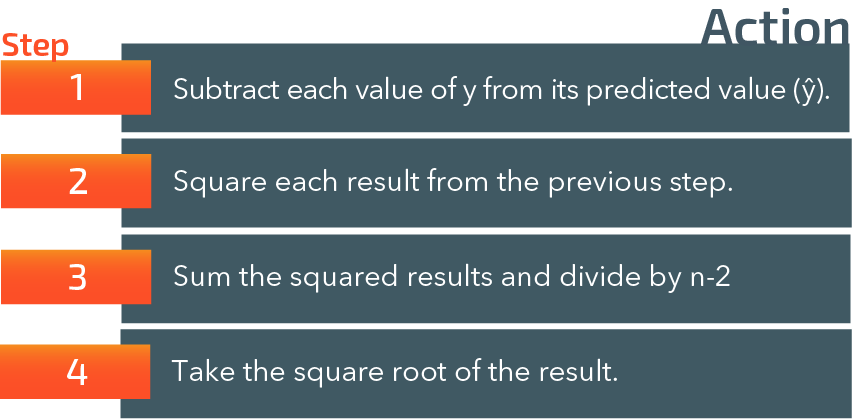Figure 14.33: Calculation steps for the standard error of the estimate

The following example illustrates these steps for our data on caloric intake and weight loss in a sample of 10 men. For clarity, we have illustrated the results for steps 1 and 2 in the two columns on the right-hand side of the table.

Example: Caloric Intake and Weight Loss

We can then apply steps 3 and 4 of the formula:

Interpretation: The standard error for the relationship between caloric intake and weight loss is 1.55.

14.26

The standard error of the estimate is a summary statistic that expresses our overall degree of error in predicting values of x based on values of y.

A

True

B

False

Use the sliders in the demonstration below to try and minimize the residual error in the linear model.This Content is Locked
Only a limited preview of this text is available. You'll need to sign up to Top Hat, and be a verified professor to have full access to view and teach with the content.

## Case Study: Do Guns Make a Nation Safer?

The debate over gun ownership in the United States has continued for over 200 years. Does their presence make people safer? One side of the debate contends that mental illness (rather than the availability of guns themselves) is the driving force behind many gun deaths, typified by several high-profile mass shootings across the U.S. in recent years. If that is the case, guns would in most cases serve to help people protect themselves. The other side of the debate maintains that reducing the number of guns, particularly high-powered assault weapons, leads to greater overall public safety. According to authors Sripal Bangalore and Franz Messerli, writing in the American Journal of Medicine (2013), there has been a dearth of systematic, comparative data to test these arguments cross-nationally. With that in mind, Bangalore and Messerli compiled data for 27 developed countries using data from the Small Arms Survey as well as the European detailed mortality database produced by the World Health Organization. They specifically included deaths from firearms due to various causes, including accidents, homicide, and suicide, as well as rates of gun ownership and rates of mental illness. Data for these countries is illustrated below: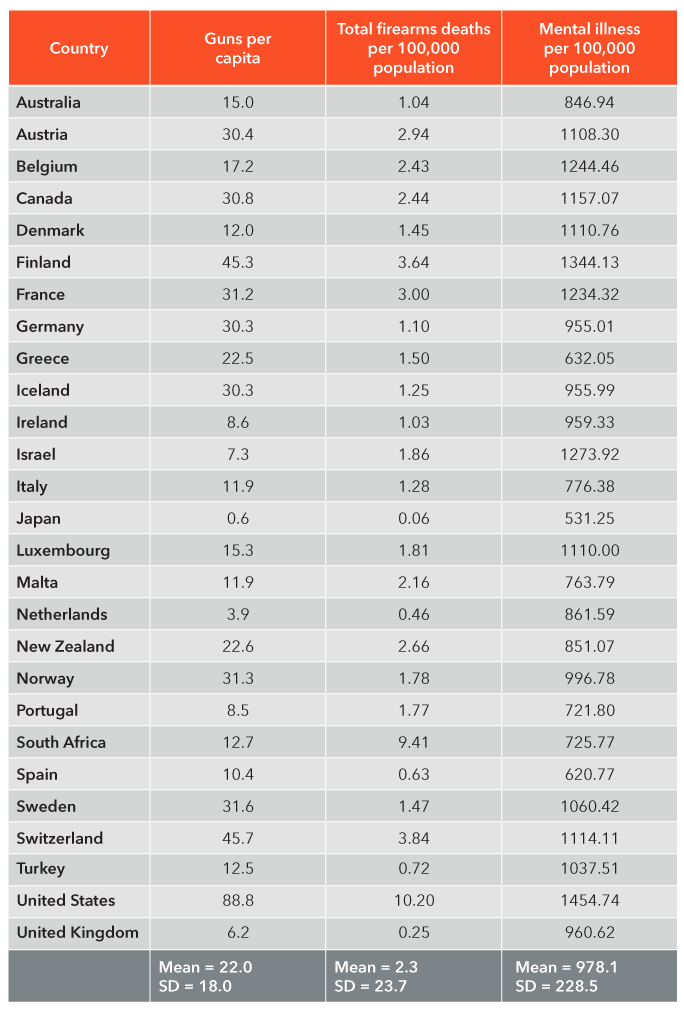Figure 14.35: Gun ownership and firearms deaths, 27 industrialized countries (2013)​​​

We can see from the table that the United States had the highest gun ownership rates (88.8 per 100 population), far outstripping any other country. There are nearly as many guns in the U.S. as there are citizens. The U.S. also had a rate of gun deaths (10.2 per 100,000 population) that far exceeded every other country except for South Africa, which followed closely behind at 9.41 per 100,000 population. (South Africa is an anomaly across developed countries in terms of its extraordinarily high crime rate, which is rooted in a complex set of unique historical and socioeconomic factors.) Japan, conversely, had the lowest rates for both of these variables (.60 and .06 respectively).

Pearson correlation analysis revealed that among these 27 countries there was a strong, statistically significant positive association between rates of gun ownership and rates of gun deaths (r = .80). South Africa was the only outlier in this association in the sense that it has a high rate of gun deaths but a relatively low rate of gun ownership. There was also a significant positive correlation (r =.52) between rates of mental illness and rates of gun deaths. Notably, the correlation between rates of gun ownership and crime rate was not statistically significant (r = .33).

Subsequent linear regression analysis was performed in order to test the extent to which gun ownership and mental illness independently predicted rates of gun deaths, i.e., taking into account each of the first two variables at the same time. The authors found that rate of gun ownership was a significant predictor of firearms deaths (p < .0001). However, mental illness was only of borderline significance (p = .05).

Their conclusion? The commonly held notion that guns make a nation safer is a myth based on their data. Regardless of the precise nature of cause and effect, in this case, higher rates of gun ownership are consistently related to higher rates of gun deaths, rather than lower ones.

(Note: Because of outliers like South Africa, the authors transformed their raw data values to log values in order to perform Pearson correlation and regression analyses, details of which can be found in their original report.)

### Case Study Question 14.01

Case Study Question 14.01

What was the key question of this study?

### Case Study Question 14.02

Case Study Question 14.02

Suppose that somebody made the following claim: “The United States has a whopping 88.8 guns per 100 people as well as 10.2 gun deaths per 100,000 population; therefore we should reduce the availability of guns.” What would be the problem with this assertion?

### Case Study Question 14.03

Case Study Question 14.03

If we were to make a scatterplot of these data for gun ownership and gun deaths, what would the pattern look like, in general?

### References

Bangalore, S. and F.H. Messerli (2013). “Gun ownership and firearm-related deaths.” American Journal of Medicine, Vol. 26:10, pp. 873-876.

Cohen, J. (1988). Statistical Power Analysis for the Behavioral Sciences (2nd edition). London: Routledge.

Gallagher, Shaun (2014). Correlated: Surprising Connections between Seemingly Unrelated Things. New York: Tarcher Perigee.

Huff (1954). How to Lie with Statistics. New York: Norton.

Judge, Timothy A., and Daniel M. Cable (2004). “The effect of physical height on workplace success and income: Preliminary test of a theoretical model”. Journal of Applied Psychology, Vol. 89, No. 3, pp. 428-441.

Levitt, Steven D., and Dubner, Stephen J. (2009). Freakonomics: A Rogue Economist Explores the Hidden Side of Everything. New York: Harper Collins.

Liu, Yi Lin et al (2016). “Association between social media use and depression among U.S. young adults.” Depression and Anxiety 33:4, pp. 323-331.

Nisbett, Richard E., and Timothy DeCamp Wilson (1977). “The Halo Effect: Evidence for unconscious alteration of judgments”. Journal of Personality and Social Psychology, Vol. 35: 4, pp. 250-256.

Patrick, Wendy (2014). “When a Mug Shot is a Glamour Shot: The Curious Case of ‘Hot’ Felon Jeremy Meeks.” https://www.psychologytoday.com/blog/why-bad-looks-good/201406/when-mug-shot-is-glamour-shot.

Tetro, Jason A. (2016). “Zika and microcephaly: Causation, correlation, or coincidence?” Microbes and Infection Vol. 18:3, pp. 167-168.

The College Board (2015). 2015 College Bound Seniors: Total Group Profile Report. Accessed online at https://secure-media.collegeboard.org/digitalServices/pdf/sat/total-group-2015.pdf.

Thorndike, E.L. (1920). “A constant error in psychological ratings.” Journal of Applied Psychology, Vol. 14:1, pp. 25-29.

Whalley, Lawrence J., and Ian Deary (2001). “Longitudinal cohort study of childhood IQ and survival up to age 76.” British Medical Journal, Vol. 322:7290, p. 819.

## Pre-Class Discussion Questions

### Class Discussion 14.01

Class Discussion 14.01

A leading herbal medicine manufacturer claims that its insomnia remedy is effective at helping people fall asleep quickly, and moreover, because it is “natural” it is safe to take in large doses. They base their claim of effectiveness upon evidence from an online survey in which visitors to their website are asked to report how long it took them to fall asleep and how much of the medicine (if any) they use on a regular basis. They claim that there is a negative association between use of their herbal remedy and amount of time spent getting to sleep (the more they take, the less time it takes to fall asleep.) What are the problems with their claims?

### Class Discussion 14.02

Class Discussion 14.02

You have access to some brand new data on race and income, and for a class final report, you decide to run regression analysis with a computer program. Your enthusiastically produce a very scientific-looking study full of numbers, including most of the statistics that you learned this term. For example, you found a strong Pearson correlation coefficient of -.70.

The marks are posted online and you are shocked and dejected to find that you received a “D” on your report, as you were so enthusiastic to show off your newfound statistical analysis skills. Before you have a chance to speak with the professor, you are trying to figure out why you got such a low grade. What might be the reason?This Content is Locked
Only a limited preview of this text is available. You'll need to sign up to Top Hat, and be a verified professor to have full access to view and teach with the content.

## Answers to Case Study Questions

### Answer to Case Study Question 14.01

The authors wanted to test the notion that the availability of guns makes a population safer, rather than less safe. A longstanding argument in the United States is that guns protect people from harm.

### Answer to Case Study Question 14.02

There is no comparison group. Comparison is crucial to understanding relationships between variables. When you encounter a claim about a group of people, always start by asking, “compared to whom?” Given the fact that the gun safety debate tends to be concentrated in the United States, it might seem easy for a researcher analyze data on gun ownership and deaths from just the U.S. But how do those death rates compare to populations with fewer guns? Do they have relatively more or relatively fewer deaths? We cannot know that for sure until we make a comparison with a population that has a different rate of gun ownership.

### Answer to Case Study Question 14.03

You could draw a straight line going from the bottom left to the top right of the scatterplot.

## Answers to Pre-Class Discussion Questions

### Answer to Class Discussion 14.01

It is problematic to assume that a correlation is causal. Ingesting a sleep medication and subsequently falling asleep does not guarantee that the medicine is what led any given person to fall asleep.

The post-hoc fallacy means “after this, therefore because of this.” Falling asleep may have occurred after taking the medicine, but it may have occurred after a variety of other things as well, such as taking a bath, reducing caffeine, or listening to relaxing music. Those other variables may have contributed to their getting to sleep far more than the herbal medicine that they took.

There are potential confounding variables that have not been accounted for, or “controlled.” For example, people who are open to take alternative medicines also tend to be more likely to be female; and females might also have an easier time falling asleep than males do. In that case, gender might be simultaneously causing both the increased use of the sleep aid as well as decreasing the amount of time that it took to fall asleep.

Experimental and control groups play a crucial role in legitimate medical research, as they isolate the effect of a stimulus (your independent variable) on a response (your dependent or outcome variable.) A key way in which they do so through the use of placebos and double-blind design, so that neither the control nor experimental group know who is actually receiving the medicine being tested. In that way, the research can establish whether a change in the independent variable really is causing a change in the dependent variable, rather than some other variable or variables.

### Answer to Class Discussion 14.02

This is a very common error – you are using a nominal variable, race, so your correlation coefficient of -.70 is meaningless. Try “drawing the arrows”. Are you able to do so? Probably not, because you would have to be able to say “As race increases, income decreases.” How can race go “up”? You cannot have “more race.”

This example goes to show that just because we learn how to calculate a statistic does not mean that it is appropriate to use in all cases.